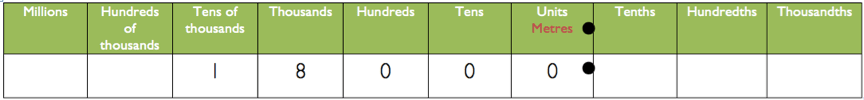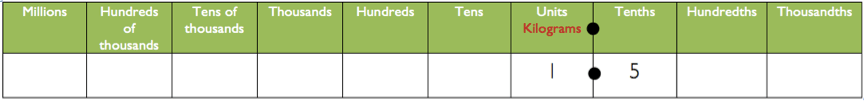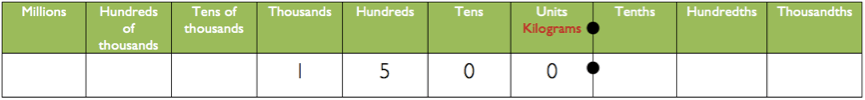# Estimating and Place Value

People working in trades or vocational training often need to estimate quantities and should always estimate before using a calculator to work out an exact amount. This often requires multiplying and dividing  by 10, 100, 1000. Many trainees find this difficult. You can use your LIteracyNumeracyPro.com place value chart to develop and practice estimation skills.

## Example 1

Let’s say that you need to find out the following: What is the total amount of timber needed for 220 lengths of 87.5m?

This is approximately 200 lengths of 90m. We can calculate this quickly as two lengths of 90m times 100.  Two lengths of 90m is 180m.To find 100 lengths of 180m shift 180 two places to the left on the place value chart. This is 18000m.

##Example 2

Here’s another example in a different context. What is the total amount of flour needed to bake 3150 loaves of bread at a local bakery? One loaf of bread uses 0.45kg of flour.

This is approximately 3000 loaves of roughly 0.5kg of flour each. We can calculate this quickly as three loaves of 0.5kg times 1000. Three loaves of 0.5kg is 1.5kg of flour.To find 1000 lots of 1.5kg shift the digits three places to the left on the place value chart.

This is 1500kg.As I said in the last post, understanding place value means understanding our number system and how it works. These examples are designed to work together with our giant Place Value Chart available from LiteracyNumeracyPro.com.Thanks once more to ALEC All-star Janet Hogan for these great examples drawn from our professional development work delivering the National Certificate in Adult Literacy and Numeracy Education (otherwise known as the NCALNE).

#Any other examples or thoughts? Let us know in the comments.## Author: Graeme Smith

Education, technology, design. Also making cool stuff...